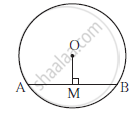Advertisement Remove all ads

# In the Adjoining Figure, Point O is the Centre of the Cirlcle, Seg Om ⊥ Chord Ab. If Om = 8cm, Ab = 12 Cm, Then Find Ob. - Geometry

In the adjoining figure, point O is the centre of the cirlcle, seg OM ⊥ chord AB. If OM = 8cm, AB = 12 cm, then find OB.Advertisement Remove all ads

#### Solution

MB = 12 × AB = 12 × 12 = 6 cm (perpendicular drawn from the centre of the circle to the chord bisects the chord)
OB^ = OM^2 + MB^2              .....................(Pythagoras thearem)
= 8^2 + 6^2
= 64 + 36 = 100
∴ OB = 10 cm

Concept: Angle Subtended by the Arc to the Point on the Circle
Is there an error in this question or solution?
Advertisement Remove all ads
Advertisement Remove all ads
Share
Notifications

View all notifications

Forgot password?